Newton's law of universal gravitation

Newton's law of universal gravitation states that every massive particle in the universe attracts every other massive particle with a force that is directly proportional to the product of their masses and inversely proportional to the square of the distance between them. (Separately it was shown that large spherically-symmetrical masses attract and are attracted as if all their mass were concentrated at their centers.) This is a general physical law derived from empirical observations by what Newton called induction. It is a part of classical mechanics and was formulated in Newton's work Philosophiae Naturalis Principia Mathematica ("the Principia"), first published on 5 July 1687. (When Newton's book was presented in 1686 to the Royal Society, Robert Hooke made a claim that Newton had obtained the inverse square law from him – see History section below.) In modern language, the law states the following:
 Every point mass attracts every single other point mass by a force pointing along the line intersecting both points. The force is proportional to the product of the two masses and inversely proportional to the square of the distance between them: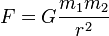$F = G \frac{m_1 m_2}{r^2}\$,where: F is the force between the masses, G is the gravitational constant, m1 is the first mass, m2 is the second mass, and r is the distance between the masses.Assuming SI units, F is measured in newtons (N), m1 and m2 in kilograms (kg), r in meters (m), and the constant G is approximately equal to 6.674×10−11 N m2 kg−2. The value of the constant G was first accurately determined from the results of the Cavendish experiment conducted by the British scientist Henry Cavendish in 1798, although Cavendish did not himself calculate a numerical value for G. This experiment was also the first test of Newton's theory of gravitation between masses in the laboratory. It took place 111 years after the publication of Newton's Principia and 71 years after Newton's death, so none of Newton's calculations could use the value of G; instead he could only calculate a force relative to another force.
Newton's law of gravitation resembles Coulomb's law of electrical forces, which is used to calculate the magnitude of electrical force between two charged bodies. Both are inverse-square laws, in which force is inversely proportional to the square of the distance between the bodies. Coulomb's Law has the product of two charges in place of the product of the masses, and the electrostatic constant in place of the gravitational constant.
Newton's law has since been superseded by Einstein's theory of general relativity, but it continues to be used as an excellent approximation of the effects of gravity. Relativity is only required when there is a need for extreme precision, or when dealing with gravitation for extremely massive and dense objects.
___________________________________________________________________________________

Vector form

Newton's law of universal gravitation can be written as a vector equation to account for the direction of the gravitational force as well as its magnitude. In this formula, quantities in bold represent vectors.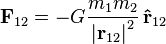$\mathbf{F}_{12} = - G {m_1 m_2 \over {\vert \mathbf{r}_{12} \vert}^2} \, \mathbf{\hat{r}}_{12}$
where
F12 is the force applied on object 2 due to object 1,
G is the gravitational constant,
m1 and m2 are respectively the masses of objects 1 and 2,
|r12| = |r2r1| is the distance between objects 1 and 2, and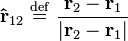$\mathbf{\hat{r}}_{12} \ \stackrel{\mathrm{def}}{=}\ \frac{\mathbf{r}_2 - \mathbf{r}_1}{\vert\mathbf{r}_2 - \mathbf{r}_1\vert}$ is the unit vector from object 1 to 2.
It can be seen that the vector form of the equation is the same as the scalar form given earlier, except that F is now a vector quantity, and the right hand side is multiplied by the appropriate unit vector. Also, it can be seen that F12 = −F21.

Gravitational field

The gravitational field is a vector field that describes the gravitational force which would be applied on an object in any given point in space, per unit mass. It is actually equal to the gravitational acceleration at that point.
It is a generalization of the vector form, which becomes particularly useful if more than 2 objects are involved (such as a rocket between the Earth and the Moon). For 2 objects (e.g. object 2 is a rocket, object 1 the Earth), we simply write r instead of r12 and m instead of m2 and define the gravitational field g(r) as:$\mathbf g(\mathbf r) = - G {m_1 \over {{\vert \mathbf{r} \vert}^2}} \, \mathbf{\hat{r}}$
so that we can write: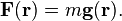$\mathbf{F}( \mathbf r) = m \mathbf g(\mathbf r).$
This formulation is dependent on the objects causing the field. The field has units of acceleration; in SI, this is m/s2.
Gravitational fields are also conservative; that is, the work done by gravity from one position to another is path-independent. This has the consequence that there exists a gravitational potential field V(r) such that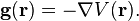$\mathbf{g}(\mathbf{r}) = - \mathbf{\nabla} V( \mathbf r).$
If m1 is a point mass or the mass of a sphere with homogeneous mass distribution, the force field g(r) outside the sphere is isotropic, i.e., depends only on the distance r from the center of the sphere. In that case$V(r) = -G\frac{m_1}{r}.$
___________________________________________________________

Problems with Newton's theory

Newton's description of gravity is sufficiently accurate for many practical purposes and is therefore widely used. Deviations from it are small when the dimensionless quantities φ/c2 and (v/c)2 are both much less than one, where φ is the gravitational potential, v is the velocity of the objects being studied, and c is the speed of light. For example, Newtonian gravity provides an accurate description of the Earth/Sun system, since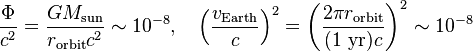$\frac{\Phi}{c^2}=\frac{GM_\mathrm{sun}}{r_\mathrm{orbit}c^2} \sim 10^{-8}, \quad \left(\frac{v_\mathrm{Earth}}{c}\right)^2=\left(\frac{2\pi r_\mathrm{orbit}}{(1\ \mathrm{yr})c}\right)^2 \sim 10^{-8}$
where rorbit is the radius of the Earth's orbit around the Sun.
In situations where either dimensionless parameter is large, then general relativity must be used to describe the system. General relativity reduces to Newtonian gravity in the limit of small potential and low velocities, so Newton's law of gravitation is often said to be the low-gravity limit of general relativity.
_____________________________________________________________________________________